## Assignment 3: Multipole Algorithm

Assigned: 3/14/95
Due: 3/23/95
Extension: 3/28/95

This assignment is designed to introduce you to more advanced uses of HPF arrays and give you the numerical feel for the multipole algorithm. Ideally, I would have asked you to build some kind of message passing tree code like the pros do. Unfortunately, I feared that this may be too difficult. Instead you are asked to write a CM Fortran code. If a small group of you would like to try to build a tree code instead, even a small toy one, please do so. You would learn a lot, and so would I! Indeed I would be glad to help.

Structure. Imagine dividing a large square into a k by k grid of cells. Further imagine subdividing each cell into a k by k grid of bodies also located on a lattice. In other words, there are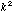bodies per cell, andcells in total giving a grand total of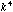cells. The following example illustrates this clearly for k = 4. There are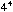= 256 bodies with sixteen bodies in each of the sixteen cells.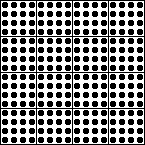1.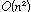calculation: Pick some random numbers (or your own favorite numbers, if you do not wish to learn to use the random number generator) for the strength of each body, and compute the potential that each body feels due to the other bodies. I think the easiest way to do this is to set a up a (2 dimensional) 3 xarray where each column corresponds to a body, and the three rows contain x, y, and q. You can then make a dynamic copy which you can cshift around so that everybody visits everybody.

Alternatively, you can loop through the columns and spread the information to every other column, by using the dynamic array to hold the spreaded columns. This may be slower because of the extra communication involved.

An approach that take more memory, but does not require loops is to use all-to-all broadcasts. You can find examples of all kinds of code in /usr/examples/... The all-to-all broadcast is buried in /usr/examples/cmssl/all-to-all . Don't be shy about trying examples and looking at the documentation.

2. One level simulation: Write a program for the following algorithm which is a hybrid fast multipole method and the direct method. First, compute the three-term expansion (up to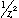) of each cell. Next compute the potentials at each point, by combining (a) the results of a direct computation for points in nearby cells and (b) using the three-term expansion for points not in nearby cells. (A cell is "nearby" if it touches at an edge or corner.) Can you estimate the number of operations as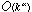, i.e. what is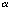?

3. Now implement a "FLIP" operation that turns a multipole expansion into a three-term local (Taylor) expansion for each cell (up to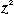). What is the operation count for this routine?

4. Modify your program so that you can handle the case where there arerandom points in each box. This means the location of the body is not centered with respect to the box, but is located elsewhere.

5. Two level simulation: So far, all we had was one level of cells: the k*k cells. (Okay, one might say we have two levels, the root representing the whole structure, and the k*k cells serving as leaves.)

Now we add a level containing k*k/4 cells formed by combing four of the previous cells into one larger cell. Therefore, what we have is the root containg the entire grid. The first level is then a (k/2) x (k/2) division into large cells. (Yes k better be even.) With 4*k*k bodies per cell. The second division divides these larger cells into the cells with k*k bodies that we worked on in parts 1, 2, 3, 4.

The first step is to compute the multipole expansion of each box at the leaf level. A shift operation combines the multipoles to the parent node. One can then work down the tree. I'll leave it to you to decide whether to do the flipping or just evaluate the multipole expansions directly.

There are a number of ways to consider implementing this program. Some ways may be easier to code, and others may run faster. In part 1, you might consider putting the strengths on a two dimensional array, and look up some fast broadcasting approaches. When learning to use a parallel machine it is up to you to figure out what runs fastest. Anyone who has touched a parallel computer knows that experimenting with different approaches is important.

For question 2, maybe one should use a 3 dimensional array, with the third axes declared serial and containing the multipole information. When you step along a serial axis, the data is local to a processor. Maybe a 5 or 6 dimensional array is more natural, or runs faster, for the other questions. Should one declare the axes serial? (Meaning that the data is local to a processor -- see the documentation) or news? (An old CM-2 term for parallel that somehow stuck.)

In your writeup, make sure it is easy to compare the numbers obtained from the direct method with the various multipole methods. Also make sure we can compare timings at each level. Run the programs for a few different k's.